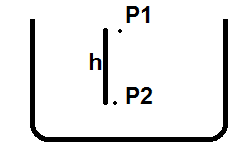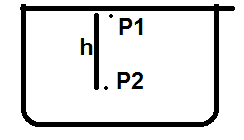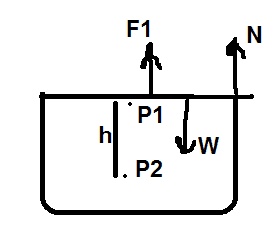# Pressure of air inside a glass

• B
• Pushoam

#### Pushoam

TL;DR Summary
How does putting a cover affect the pressure inside a glass filled with air?
Let's consider an uncovered glass. Air particles are present in the glass.$$P_1 = P_a$$ $$P_2 =P_1 +\rho gh = P_a +\rho g h$$where ##P_A## is atmospheric pressuere and ##\rho ## is air density.Now, if I cover the glass with a plastic card, then what is ## P_1##?
$$P_2 =P_1 +\rho gh$$
1) ## P_1 ## is pressure due to motion of air particles and the air particles near the cover interact with the cover and its speed may change and hence ##P_1## may be less or more than ##P_a##.

2) Following three forces are acting on the cover:a) force due to pressure ##P_1## of air particles
b) normal force N due to glass walls
c) cover's weight W

Applying Newton's first law gives,
$$P_1 A+ N = W$$ $$P_1 A = W - N$$
Now, since normal force is self-adjustable, let's take a light plastic card such that N = 0. Hence, in this case ##P_1 A = W ##.
For a plastic card with mass 20g and area 20cm2, ## P_1 = 10 Pa## which is lower than the atmospheric pressure.
So, the conclusion is: putting a cover reduces the pressure of air inside the glass. Is this correct?

So, the conclusion is: putting a cover reduces the pressure of air inside the glass. Is this correct?
No.
There will be a hydrostatic difference in pressure due to the thickness of the card, but that difference will be overcome by the density of the card, which is resting on the glass.

•russ_watters
No.
There will be a hydrostatic difference in pressure due to the thickness of the card, but that difference will be overcome by the density of the card, which is resting on the glass.
And/or the card will bend down/inward due to its own weight and the resulting pressure in the glass will be higher than atmospheric.

Define Pa as the atmospheric pressure at the top edge, inside the glass.
The following four forces are acting on a cover of thickness; t
a) force due to pressure of air from below; A·Pa
b) force due to pressure of air from above; A·(Pa - ρ·g·t)
c) cover's weight; W
d) normal force upwards due to glass wall; N
N + A·Pa = W + A·(Pa - ρ·g·t)
N = W - ρ·g·t
ρ·g·t
is the buoyancy of the card